login  home  contents  what's new  discussion  bug reports help  links  subscribe  changes  refresh  edit

FriCAS inherited from Axiom implementation of Risch algorithm for elementary integration. It can handle both easy cases

fricas
(1) -> integrate(x*exp(x^2), x)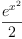(1)
Type: Union(Expression(Integer),...)
fricas
integrate(exp(1/x^2)/x^3, x)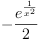(2)
Type: Union(Expression(Integer),...)

and some which are hard for simpler algorithms. For example Maxma 5.45.1 can not handle

fricas
integrate(sqrt(x^2+1)/(x^3+1), x)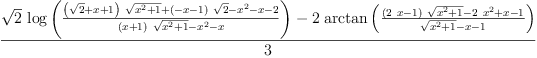(3)
Type: Union(Expression(Integer),...)

fricas
)set output tex off

fricas
)set output algebra on
integrate(sqrt(x^2+1)/(x^4+1), x)
(4)
+----------+     +----------+     +------+
| +---+          | +---+          | 2         +---+    2
- \|\|- 1  - 1 log(\|\|- 1  - 1  - x\|x  + 1  - \|- 1  + x )
+
+------------+        +------+    +------------+
|   +---+             | 2         |   +---+         +---+    2
- \|- \|- 1  - 1 log(- x\|x  + 1  + \|- \|- 1  - 1  + \|- 1  + x )
+
+------------+        +------+    +------------+
|   +---+             | 2         |   +---+         +---+    2
\|- \|- 1  - 1 log(- x\|x  + 1  - \|- \|- 1  - 1  + \|- 1  + x )
+
+----------+       +----------+     +------+
| +---+            | +---+          | 2         +---+    2
\|\|- 1  - 1 log(- \|\|- 1  - 1  - x\|x  + 1  - \|- 1  + x )
/
4
Type: Union(Expression(Integer),...)
fricas
)set output algebra off

fricas
)set output tex on

Default integrators in Mathematica 12.0 and Maple 15 can not handle

fricas
integrate(((exp(x)-x^2+2*x)/(x^2*(exp(x)+x)^2))*exp((x^2-1)/x+1/(exp(x)+x)), x)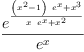(4)
Type: Union(Expression(Integer),...)

Default integrators in Maple 15 and Maxima 5.45.1 can not handle

fricas
integrate(x^6/sqrt((x^7+1)*(x^7+2)), x)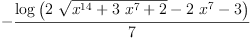(5)
Type: Union(Expression(Integer),...)

FriCAS fixed several bugs in Axiom implementation, for example

fricas
integrate(cos(x)^n*sin(x)^(-n-2), x)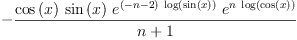(6)
Type: Union(Expression(Integer),...)
fricas
integrate(exp(asec(x))/x^2, x)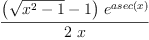(7)
Type: Union(Expression(Integer),...)

work now but used to return unevaluated result.

Risch algorithm can handle large class of integrands involving special functions

fricas
f := D(1/(1 + ellipticE(x^2+a, m)), x)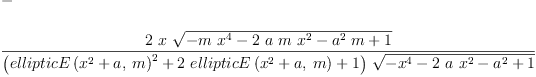(8)
Type: Expression(Integer)
fricas
integrate(f, x)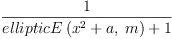(9)
Type: Union(Expression(Integer),...)
fricas
f := D(1/(Ei(x)-Ei(x-1)), x)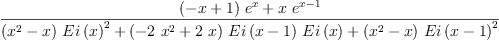(10)
Type: Expression(Integer)
fricas
integrate(f, x)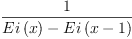(11)
Type: Union(Expression(Integer),...)
fricas
f := D(1/(Si(x+1)+Ci(x-1)), x)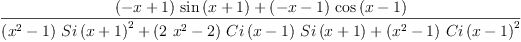(12)
Type: Expression(Integer)
fricas
integrate(f, x)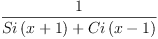(13)
Type: Union(Expression(Integer),...)
fricas
f := D(1/(Si(Ci(x)^2+1)+Ci(Ci(x)^2-1)), x)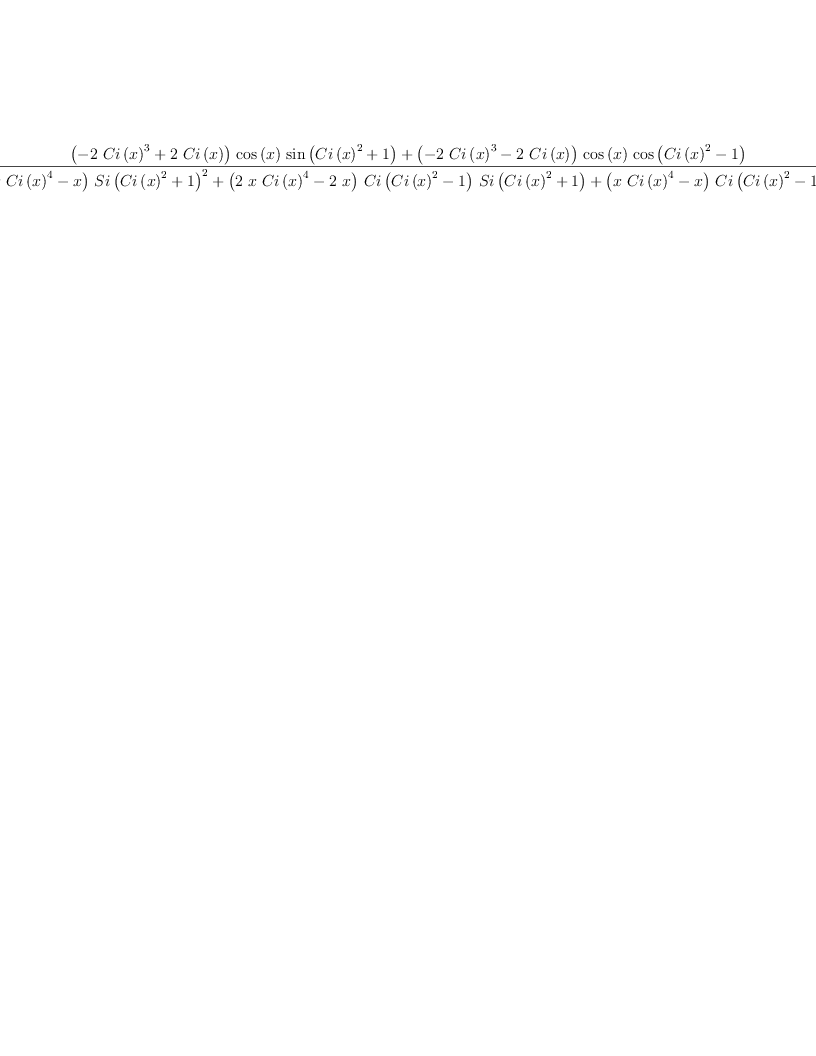(14)
Type: Expression(Integer)
fricas
integrate(f, x)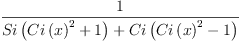(15)
Type: Union(Expression(Integer),...)
fricas
f := D(log(Si(Ci(x)^2+1)+Ci(Ci(x)^2-1)), x)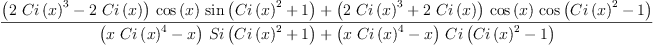(16)
Type: Expression(Integer)
fricas
integrate(f, x)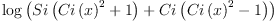(17)
Type: Union(Expression(Integer),...)

Alas, the following does not work

fricas
f := D(1/(1 + besselJ(m, x^2+a)), x)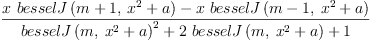(18)
Type: Expression(Integer)
fricas
integrate(f, x)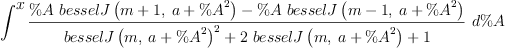(19)
Type: Union(Expression(Integer),...)

Risch algorithm wants to find elementary integral. For many functions integrals can be written only in terms of special function. FriCAS now contains extensions which can handle some of them, see FriCASSpecialIntegration.

For more elementary examples see FriCASTimofeev1, FriCASTimofeev37, FriCASTimofeev5, FriCASTimofeev9.

Examples involving exponential function from Albert Rich testsuite RichExp1, RichExp2.

integrate(exp(x-x^2), x) --mariuszi28cg, Mon, 25 Sep 2017 20:48:15 +0000 reply
fricas
integrate(exp(x-x^2), x)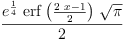(20)
Type: Union(Expression(Integer),...)

Most probably non-elementary, but fricas fails here as of 16.10.22 --xak, Sun, 16 Oct 2022 17:56:19 +0000 reply
fricas
integrate(sqrt((sin(x)^2 + x)/exp(x)),x)
>> Error detected within library code:
integrate: implementation incomplete (has polynomial part)

Dr. Waldemar Hebisch kindly proved this one to be non-elementary when we spoke --xak, Sun, 16 Oct 2022 17:59:04 +0000 reply
fricas
integrate(sqrt(sin(x)+x),x)
>> Error detected within library code:
integrate: implementation incomplete (has polynomial part)

 Subject:   Be Bold !! ( 14 subscribers )